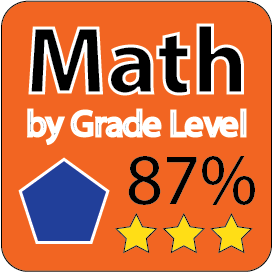Back to Mr. Anker Testsand maybe the grade above yours!

Teachers & Parents: There are just 10 questions in each activity.
It is recommended that students complete and master
each activity with a 9 or 10 before moving on to the next one.

 2 Math A 2 Math B 2 Math C 2 Math D

 3 Math A 3 Math B 3 Math C 3 Math D
 3 Math E 3 Math F 3 Math G 3 Math H
 3 Math I 3 Math J 3 Math K 3 Math L
 3 Math M 3 Math N 3 Math O 3 Math P
 3 Math Q

 4 Math A 4 Math B Probability 1 (Not Yet Ready) Probability 2 (Not Yet Ready)

Twisted Word Problems (4th-5th Grade Level)
 Twisted Word Problems 1 Twisted Word Problems 2 Twisted Word Problems 3 Twisted Word Problems 4

 5 Math A 5 Math B 5 Math C 5 Math D
 5 Math E 5 Math F 5 Math G 5 Math H
 5 Math I 5 Math J